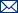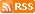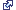BCO-DMO ERDDAP Accessing BCO-DMO data log in     Brought to you by BCO-DMO

# ERDDAP > tabledap > SubsetDataset Title: Time series HPLC pigment data as measured by the Bermuda Biological Research Station (BBRS) from B/O Hermano Gines cruises CAR-002 to CAR-030 in the CARIACO basin from 1995-12-13 through 1998-04-21 (CARIACO Ocean Time-Series Program)Institution: BCO-DMO   (Dataset ID: bcodmo_dataset_3293) Information: Summary| License| ISO 19115 | Metadata | Background| Data Access Form | Files | Make a graph

Select a subset:      (Current number of distinct combinations of matching data: 1)
Make as many selections as you want, in any order. Each selection changes the other options (and the map and data below) accordingly.

 Analyzed_by= (ANY) Bermuda biological Research Station (Cathy Rathburn)  unitless 1 option: Bermuda biological Research Station (Cathy Rathburn) lon_n= (ANY) -64.67  decimal degrees 1 option: -64.67 lat_n= (ANY) 10.5  decimal degrees 1 option: 10.5 depth_bottom= (ANY) 1380  meters (m) 1 option: 1380 Tot_Chl_c= (ANY) NaN  miligrams per meter cubed (mg/m^3) 1 option: alpha_beta_Car= (ANY) NaN  miligrams per meter cubed (mg/m^3) 1 option: MV_Chl_a= (ANY) NaN  miligrams per meter cubed (mg/m^3) 1 option: DV_Chl_a= (ANY) NaN  miligrams per meter cubed (mg/m^3) 1 option: Chl_a_allom= (ANY) NaN  miligrams per meter cubed (mg/m^3) 1 option: Chl_a_prime= (ANY) NaN  miligrams per meter cubed (mg/m^3) 1 option: MV_Chl_b= (ANY) NaN  miligrams per meter cubed (mg/m^3) 1 option: DV_Chl_b= (ANY) NaN  miligrams per meter cubed (mg/m^3) 1 option: Chl_c1= (ANY) NaN  miligrams per meter cubed (mg/m^3) 1 option: Chl_c2= (ANY) NaN  miligrams per meter cubed (mg/m^3) 1 option: Neo= (ANY) NaN  miligrams per meter cubed (mg/m^3) 1 option: Viola= (ANY) NaN  miligrams per meter cubed (mg/m^3) 1 option: Phytin_a= (ANY) NaN  miligrams per meter cubed (mg/m^3) 1 option: Phide_a= (ANY) NaN  miligrams per meter cubed (mg/m^3) 1 option: Anth= (ANY) NaN  miligrams per meter cubed (mg/m^3) 1 option: Gyro= (ANY) NaN  miligrams per meter cubed (mg/m^3) 1 option: TChl= (ANY) NaN  miligrams per meter cubed (mg/m^3) 1 option: PPC= (ANY) NaN  miligrams per meter cubed (mg/m^3) 1 option: PSC= (ANY) NaN  miligrams per meter cubed (mg/m^3) 1 option: PSP= (ANY) NaN  miligrams per meter cubed (mg/m^3) 1 option: Tcar= (ANY) NaN  miligrams per meter cubed (mg/m^3) 1 option: Tacc= (ANY) NaN  miligrams per meter cubed (mg/m^3) 1 option: Tpg= (ANY) NaN  miligrams per meter cubed (mg/m^3) 1 option: DP= (ANY) NaN  miligrams per meter cubed (mg/m^3) 1 option: Tacc_TChla= (ANY) NaN  unitless 1 option: PSC_Tcar= (ANY) NaN  unitless 1 option: PPC_Tcar= (ANY) NaN  unitless 1 option: TChl_Tcar= (ANY) NaN  unitless 1 option: PPC_Tpg= (ANY) NaN  unitless 1 option: PSP_Tpg= (ANY) NaN  unitless 1 option: TChla_Tpg= (ANY) NaN  unitless 1 option: mPF= (ANY) NaN  unitless 1 option: nPF= (ANY) NaN  unitless 1 option: pPF= (ANY) NaN  unitless 1 option:

View:      Map of All Related DataDistinct Data CountsDistinct DataRelated Data CountsRelated DataMap of All Related Data(Refine the map and/or download the image)

To view the map, check View : Map of All Related Data above.

WARNING: This may involve lots of data. For some datasets, this may be slow. Consider using this only when you need it and have selected a small subset of the data.

Distinct Data CountsTo view the counts of distinct combinations of the variables listed above,
check View : Distinct Data Counts above and select a value for one of the variables above.

Distinct Data(Metadata)    (Refine the data subset and/or download the data)

Analyzed_by lon_n lat_n depth_bottom Tot_Chl_c alpha_beta_Car MV_Chl_a DV_Chl_a Chl_a_allom Chl_a_prime MV_Chl_b DV_Chl_b Chl_c1 Chl_c2 Neo Viola Phytin_a Phide_a Anth Gyro TChl PPC PSC PSP Tcar Tacc Tpg DP Tacc_TChla PSC_Tcar PPC_Tcar TChl_Tcar PPC_Tpg PSP_Tpg TChla_Tpg mPF nPF pPF
unitless decimal degrees decimal degrees meters (m) miligrams per meter cubed (mg/m^3) miligrams per meter cubed (mg/m^3) miligrams per meter cubed (mg/m^3) miligrams per meter cubed (mg/m^3) miligrams per meter cubed (mg/m^3) miligrams per meter cubed (mg/m^3) miligrams per meter cubed (mg/m^3) miligrams per meter cubed (mg/m^3) miligrams per meter cubed (mg/m^3) miligrams per meter cubed (mg/m^3) miligrams per meter cubed (mg/m^3) miligrams per meter cubed (mg/m^3) miligrams per meter cubed (mg/m^3) miligrams per meter cubed (mg/m^3) miligrams per meter cubed (mg/m^3) miligrams per meter cubed (mg/m^3) miligrams per meter cubed (mg/m^3) miligrams per meter cubed (mg/m^3) miligrams per meter cubed (mg/m^3) miligrams per meter cubed (mg/m^3) miligrams per meter cubed (mg/m^3) miligrams per meter cubed (mg/m^3) miligrams per meter cubed (mg/m^3) miligrams per meter cubed (mg/m^3) unitless unitless unitless unitless unitless unitless unitless unitless unitless unitless
Bermuda biological Research Station (Cathy Rathburn) -64.67 10.5 1380

In total, there are 1 rows of distinct combinations of the variables listed above. All of the rows are shown above.
To change the maximum number of rows displayed, change View : Distinct Data above.

Related Data CountsTo view the related data counts,
check View : Related Data Counts above and select a value for one of the variables above.

WARNING: This may involve lots of data. For some datasets, this may be slow. Consider using this only when you need it and have selected a small subset of the data.

Related Data(Metadata)    (Refine the data subset and/or download the data)

To view the related data, change View : Related Data above.

WARNING: This may involve lots of data. For some datasets, this may be slow. Consider using this only when you need it and have selected a small subset of the data.

ERDDAP, Version 2.02